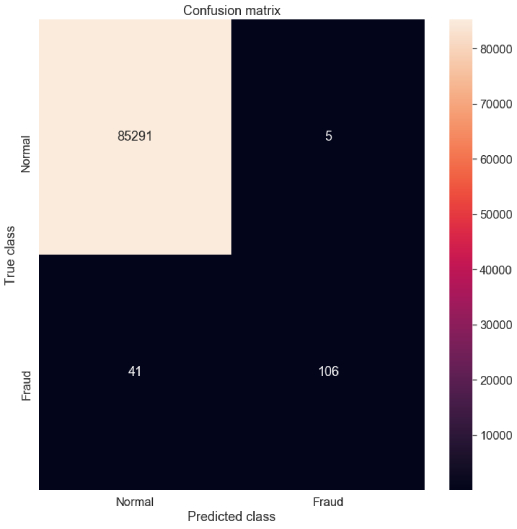• +91-9872993883
• +91-8283824812
• info@ris-ai.com

# Credit Card Fraud Detection¶

Hello guys here we will see how we can predict the creditcard fraud through machine learning model. The challenge is to recognize fraudulent credit card transactions so that the customers of credit card companies are not charged for items that they did not purchase.

In :
import numpy as np
import pandas as pd
import matplotlib.pyplot as plt
import seaborn as sns
from matplotlib import gridspec


here we read our given dataset

In :
data = pd.read_csv("creditcard.csv")

Out:
Time V1 V2 V3 V4 V5 V6 V7 V8 V9 ... V21 V22 V23 V24 V25 V26 V27 V28 Amount Class
0 0.0 -1.359807 -0.072781 2.536347 1.378155 -0.338321 0.462388 0.239599 0.098698 0.363787 ... -0.018307 0.277838 -0.110474 0.066928 0.128539 -0.189115 0.133558 -0.021053 149.62 0
1 0.0 1.191857 0.266151 0.166480 0.448154 0.060018 -0.082361 -0.078803 0.085102 -0.255425 ... -0.225775 -0.638672 0.101288 -0.339846 0.167170 0.125895 -0.008983 0.014724 2.69 0
2 1.0 -1.358354 -1.340163 1.773209 0.379780 -0.503198 1.800499 0.791461 0.247676 -1.514654 ... 0.247998 0.771679 0.909412 -0.689281 -0.327642 -0.139097 -0.055353 -0.059752 378.66 0
3 1.0 -0.966272 -0.185226 1.792993 -0.863291 -0.010309 1.247203 0.237609 0.377436 -1.387024 ... -0.108300 0.005274 -0.190321 -1.175575 0.647376 -0.221929 0.062723 0.061458 123.50 0
4 2.0 -1.158233 0.877737 1.548718 0.403034 -0.407193 0.095921 0.592941 -0.270533 0.817739 ... -0.009431 0.798278 -0.137458 0.141267 -0.206010 0.502292 0.219422 0.215153 69.99 0

5 rows × 31 columns

In :
print(data.shape)

(284807, 31)


#### Time to explain the data we are dealing with. ¶

Only 0.17% fraudulent transaction out all the transactions. The data is highly Unbalanced.

In :
fraud = data[data['Class'] == 1]
valid = data[data['Class'] == 0]
outlierFraction = len(fraud)/float(len(valid))
print(outlierFraction)
print('Fraud Cases: {}'.format(len(data[data['Class'] == 1])))
print('Valid Transactions: {}'.format(len(data[data['Class'] == 0])))

0.0017304750013189597
Fraud Cases: 492
Valid Transactions: 284315

In :
print("Amount details of the fraudulent transaction")
fraud.Amount.describe()

Amount details of the fraudulent transaction

Out:
count     492.000000
mean      122.211321
std       256.683288
min         0.000000
25%         1.000000
50%         9.250000
75%       105.890000
max      2125.870000
Name: Amount, dtype: float64
In :
print("details of valid transaction")
valid.Amount.describe()

details of valid transaction

Out:
count    284315.000000
mean         88.291022
std         250.105092
min           0.000000
25%           5.650000
50%          22.000000
75%          77.050000
max       25691.160000
Name: Amount, dtype: float64

## Plotting the Correlation Matrix¶

The correlation matrix graphically gives us an idea of how features correlate with each other and can help us predict what are the features that are most relevant for the prediction.

In the HeatMap we can clearly see that most of the features do not correlate to other features but there are some features that either has a positive or a negative correlation with each other.

For example, V2 and V5 are highly negatively correlated with the feature called Amount. We also see some correlation with V20 and Amount.

In :
# Correlation matrix
corrmat = data.corr()
fig = plt.figure(figsize = (12, 12))
sns.heatmap(corrmat, vmax = .8, square = True)
plt.show()In :
# dividing the X and the Y from the dataset
X = data.drop(['Class'], axis = 1)
Y = data["Class"]
print(X.shape)
print(Y.shape)
# getting just the values for the sake of processing
# (its a numpy array with no columns)
xData = X.values
yData = Y.values

(284807, 30)
(284807,)


We will be dividing the dataset into two main groups. One for training the model and the other for Testing our trained model’s performance.

In :
# Using Skicit-learn to split data into training and testing sets
from sklearn.model_selection import train_test_split
# Split the data into training and testing sets
xTrain, xTest, yTrain, yTest = train_test_split(xData, yData, train_size = 0.7, random_state = 0)


here we are using RandomForest model

In :
# Building the Random Forest Classifier (RANDOM FOREST)
from sklearn.ensemble import RandomForestClassifier
# random forest model creation
rfc = RandomForestClassifier()
rfc.fit(xTrain, yTrain)
# predictions
yPred = rfc.predict(xTest)

/home/webtunix/.local/lib/python3.5/site-packages/sklearn/ensemble/forest.py:245: FutureWarning: The default value of n_estimators will change from 10 in version 0.20 to 100 in 0.22.
"10 in version 0.20 to 100 in 0.22.", FutureWarning)

In :
from sklearn.metrics import f1_score, accuracy_score ,precision_score
from sklearn.metrics import confusion_matrix

n_outliers = len(fraud)
n_errors = (yPred != yTest).sum()

acc = accuracy_score(yTest, yPred)
print("The accuracy is {}".format(acc))

prec = precision_score(yTest, yPred)
print("The precision is {}".format(prec))

f1 = f1_score(yTest, yPred)
print("The F1-Score is {}".format(f1))

The accuracy is 0.9994616293903538
The precision is 0.954954954954955
The F1-Score is 0.8217054263565892


Visulalizing the Confusion Matrix

In :
# printing the confusion matrix
LABELS = ['Normal', 'Fraud']
conf_matrix = confusion_matrix(yTest, yPred)
plt.figure(figsize =(12, 12))
sns.heatmap(conf_matrix, xticklabels = LABELS,
yticklabels = LABELS, annot = True, fmt ="d");
plt.title("Confusion matrix")
plt.ylabel('True class')
plt.xlabel('Predicted class')
plt.show()In [ ]: